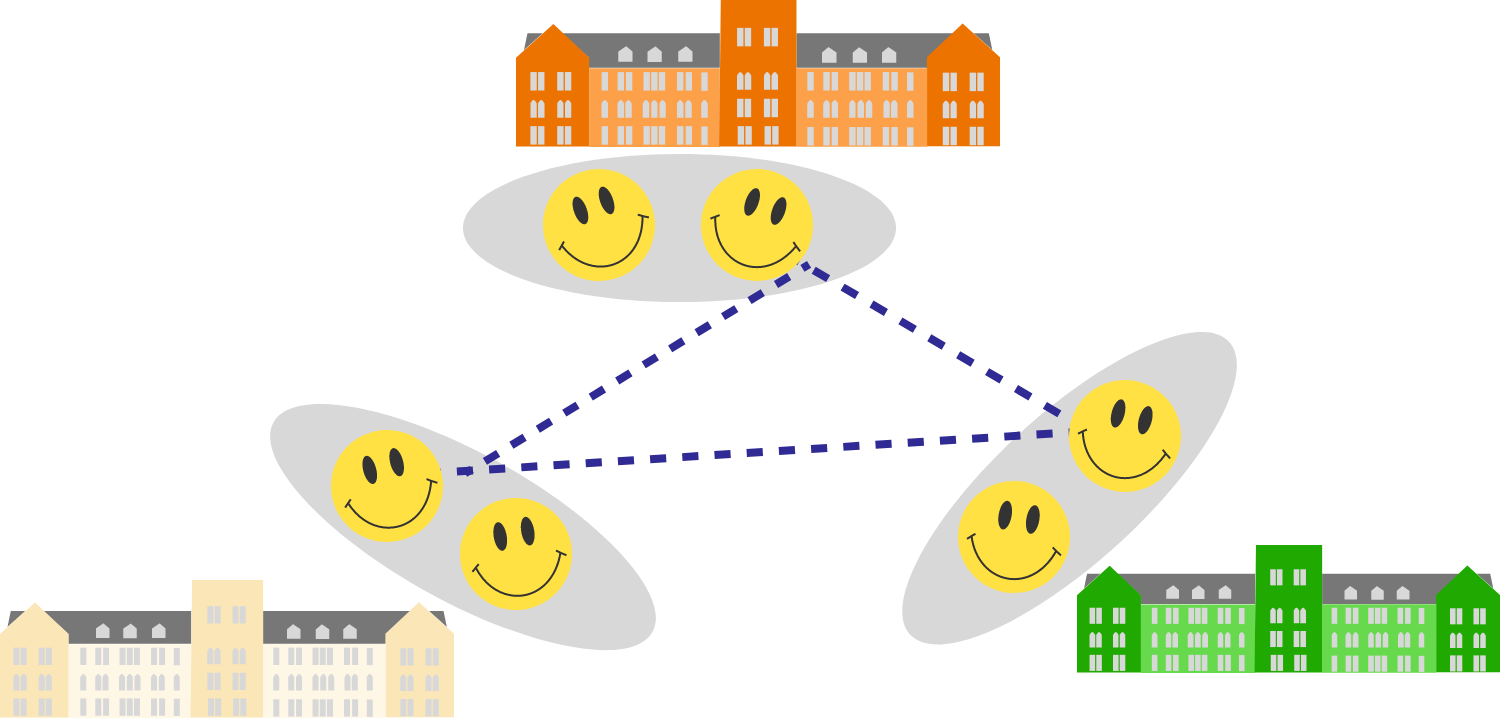Probability Level 3

There are three schools, each with exactly 2017 students. Every student is friends with at least 2018 students from the other two schools.

Does there exist a group of 3 students--one from each of the three schools--that are all pairwise friends?Note: If $A$ is friends with $B,$ then $B$ is friends with $A.$

×